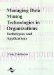# Introduction

 < Day Day Up >

Frontier or benchmark estimation models were first discussed by Aigner and Chu (1968), who fitted a Cobb-Douglas industry production function to data on production levels and factors. Here the Cobb-Douglas model was proposed as the best possible, frontier or benchmark model for the data. Observed production levels were modeled by subtracting nonnegative errors or inefficiency shortfalls from the frontier. More generally, such models may be called "Frontier Regression Models." They seek to explain boundary, frontier or optimal behavior rather than average behavior as in ordinary regression models. Such a model may also be called a ceiling model as it lies above all the observations. (The opposite case is similarly called a floor model.) Ordinary regression is one of the most important tools for data mining. Frontier models may be desirable alternatives in some circumstances. In this chapter, we discuss frontier regression models and compare them to ordinary regression models. We also propose guidelines for when to choose between them.

There are a related class of models called stochastic frontier estimation (SFE) models. These are modifications of the pure frontier method first considered separately by Meeusen and van den Broeck (1977) and Aigner, Lovell and Schmidt (1977). Here actual performance is modeled as the frontier model plus an error term composed of two parts. The first error part is normally distributed with mean zero. It is usually justified as accounting for uncertainty in the frontier model. The second error part is a nonnegative one, representing a measure of inefficiency error or deviation from the efficient frontier as in the pure frontier model. This term is also called the inefficiency effect in Coelli, Prasada Rao and Battese (1998). The Aigner et al. (1977) method assumes that such nonnegative inefficiencies are distributed as half-normal. This permits the distribution of the total error to be specified and its parameters to be estimated by the maximum likelihood method. Stevenson (1980) extended that method to permit assumption of truncated normal and gamma distributions. However, Ritter and Léopold (1997) have found that such models are difficult to accurately estimate. Recently, Troutt, Hu and Shanker (2001) have pointed out theoretical problems in maximizing the likelihood function for such models. In that research, it was found that the likelihood function is U-shaped. One end corresponds to assuming a pure frontier model and the other corresponds to a pure ordinary least squares regression model. Thus, that research suggests that the maximum likelihood principle will choose one of those end point cases and not a mixed or SFE type model. We therefore concentrate on pure frontier models in this chapter.

 < Day Day Up >Managing Data Mining Technologies in Organizations: Techniques and Applications
ISBN: 1591400570
EAN: 2147483647
Year: 2003
Pages: 174